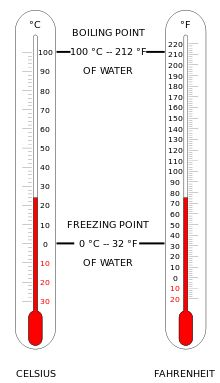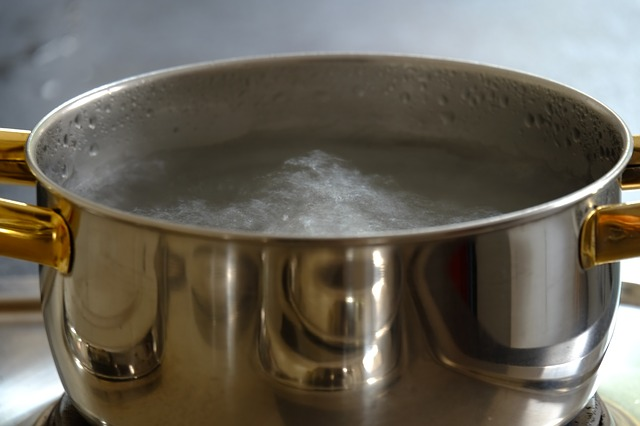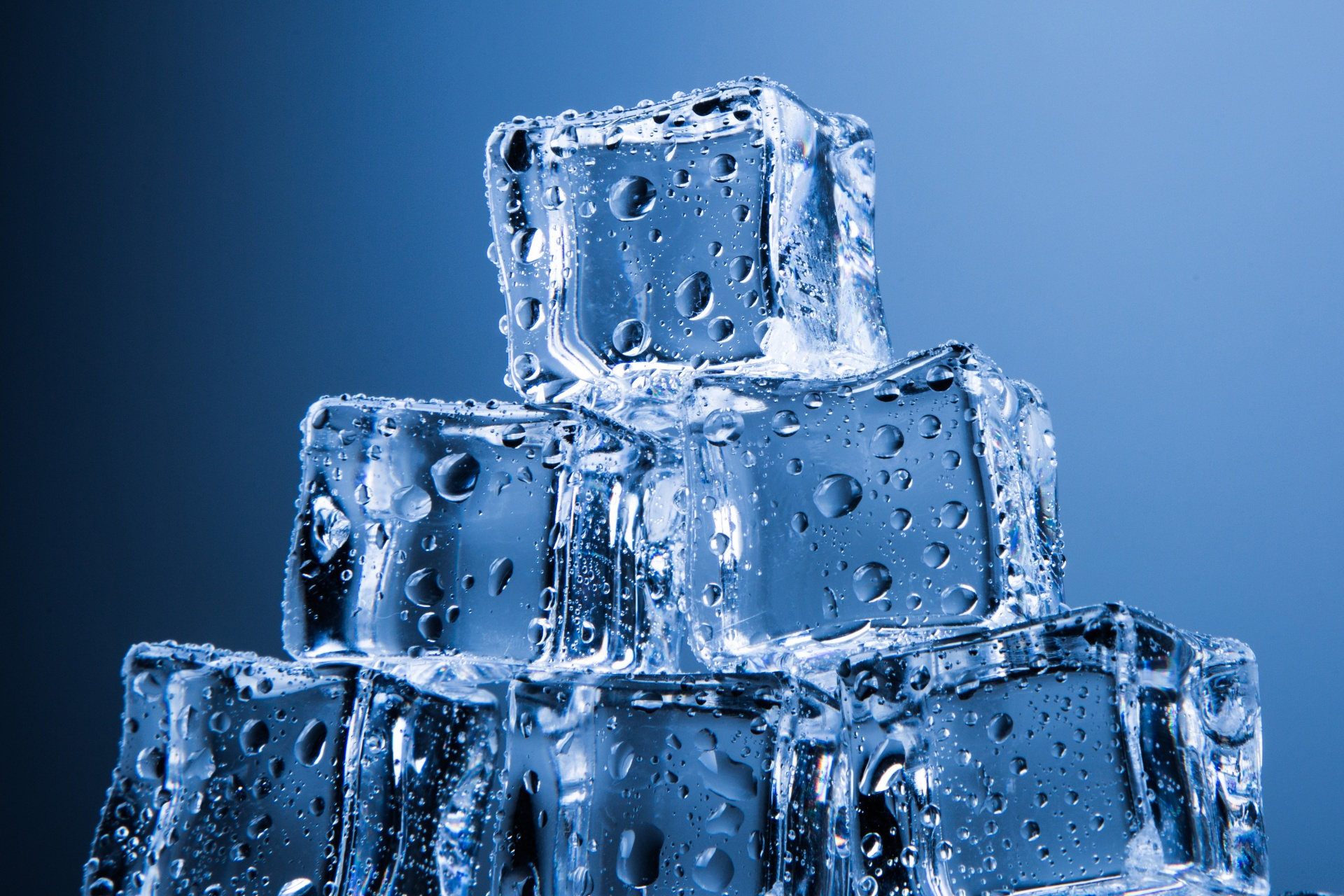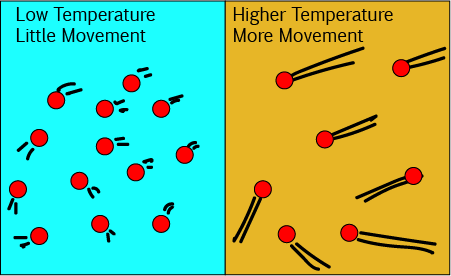# Converting Temperatures

## Converting Between Celsius and FahrenheitThe two temperature scales used most commonly in everyday life are the Celsius and Fahrenheit scales. Most countries report temperatures according to the Celsius scale, but some, most notably the US use the Fahrenheit scale. Your grandparents may also have grown up with the Fahrenheit scale because we didn't start to use the Celsius scale officially in Australia until 1970 (1976 in New Zealand).

The chances are that, some time in your life, you'll hear someone talking about temperatures in degrees Fahrenheit, so it's worthwhile learning to convert between the two scales.

• To convert from ${}^o F$ to ${}^o C$: take away $32$, and then multiply by $\dfrac{5}{9}$.
• To convert from ${}^o C$ to ${}^o F$: multiply by $\dfrac{9}{5}$, and then add on $32$.

### Some Common Temperatures and their Equivalents on both Scales.

${}^\circ \text{F}$ ${}^\circ \text{C}$ Description
$0$ $32$ Water freezes
$212$ $100$ Water boils
$104$ $40$ Lukewarm
$120$ $49$ Safe temperature for hot water
$98.6$ $37$ Normal human body temperature
$100$ $37.8$ Very hot day
$86$ $30$ Nice day for the beach
$70$ $21$ Standard room temperature
$50$ $10$ Cold day
$0$ $-18$ Really cold day
$-40$ $-40$ Ridiculously cold day. This is where the two scales match up.

### Why the Conversion Works

The Fahrenheit and Celsius scales both measure the same thing, but their degrees have different sizes and they start at different places:Water boils at $100^\circ \text{C}$ (on the Celsius, or metric scale) and $212^\circ \text{F}$.Water freezes at $0^\circ \text{C}$ (on the Celsius, or metric scale) and $32^\circ \text{F}$.

Because the two scales start at different numbers, we need to add or subtract $32$ in our conversions.

The size of the degrees is different in each scale (the Celsius scale takes $100^\circ$ to move from water freezing to water boiling, while the Fahrenheit scale takes $180^\circ$ to move from water freezing to water boiling), so we need to multiply the Celsius degrees by $\dfrac{180}{100} = \dfrac{9}{5}$ to convert them to Fahrenheit degrees.

Combining these two steps gives us our conversion formulas:

Celsius to Fahrenheit: Celsius Temperature $\times \dfrac{9}{5} + 32 =$ Fahrenheit Temperature.
Fahrenheit to Celsius: (Fahrenheit Temperature $- 32) \times \dfrac{5}{9} =$ Celsius Temperature.

Let's try a few examples.

The temperature today was $32^\circ \text{C}$. In Fahrenheit, that is $32 \times \dfrac{9}{5} + 32 = 89.6^\circ \text{F}$.

When we visited our family in Canada, the temperature was $-20^\circ \text{C}$. In Fahrenheit, that is $-20 \times \dfrac{9}{5} + 32 = -4^\circ \text{F}$.

While we were in Canada, we saw weather reports from the US. They said the temperature was $16^\circ \text{F}$. In Celsius, that is $(16 - 32)\times \dfrac{5}{9} = -8.9^\circ\text{ C}$.

Our weather station sometimes has a glitch and reports the temperature in Fahrenheit. At the moment, it is reading $73.4^\circ \text{ F}$. In Celsius, that is $(73.4 - 32) \times \dfrac{5}{9} = 23^\circ \text{ C}$.

## A Third Temperature Scale Used by Scientists

Did you know that the SI unit for temperature is not degrees Celsius? It is Kelvin. The size of the Kelvin unit is exactly the same as one degree Celsius, but the two scales start at different points.

$0^\circ \text{ C}$ is actually $273.16 \text{ K}$. There are no "degrees" in the unit for the Kelvin scale.

The temperature of $0 \text{ K}$ is called absolute zero, but it can never be reached. It represents the absence of all heat.

The temperature of an object measures how fast its particles are moving. Cool temperatures correspond to slowly moving particles, and warm temperatures correspond to more quickly moving particles.Theoretically, a temperature of $0 \text{ K}$ is the temperature at which the particles of the object stop moving altogether.

It is easy to convert from degrees Celsius to Kelvin: just add $273.16$ to your Celsius temperature. To go back the other way, subtract $273.16$ from your Kelvin temperature to give a temperature in degrees Celsius.

### Description

• Metric numbers
• Speed
• Conversions

and several other topics

### Audience

Year 10 or higher, some chapters are suitable for Year 8+ students

### Learning Objectives

Learn about measurement and related topics

Author: Subject Coach
You must be logged in as Student to ask a Question.